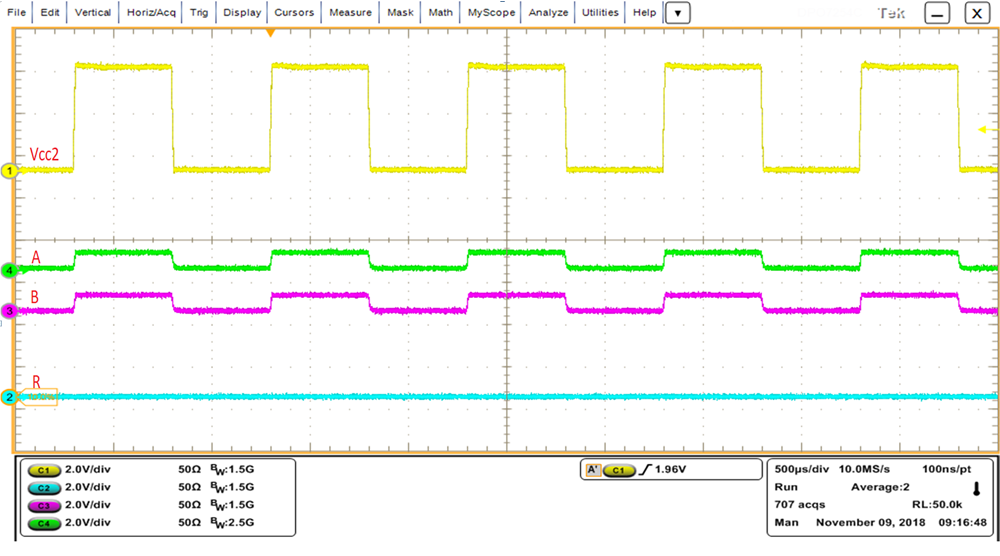JAJSGA9C September   2018  – September 2019

PRODUCTION DATA.

1. 特長
2. アプリケーション
3. 概要
4. 改訂履歴
5. 概要 (続き)
6. Pin Configuration and Functions
7. Specifications
8. Parameter Measurement Information
9. Detailed Description
1. 9.1 Overview
2. 9.2 Functional Block Diagram
3. 9.3 Feature Description
4. 9.4 Device Functional Modes
10. 10Application and Implementation
1. 10.1 Application Information
2. 10.2 Typical Application
1. 10.2.1 Design Requirements
2. 10.2.2 Detailed Design Procedure
11. 11Power Supply Recommendations
12. 12Layout
1. 12.1 Layout Guidelines
2. 12.2 Layout Example
13. 13デバイスおよびドキュメントのサポート
14. 14メカニカル、パッケージ、および注文情報

• DBQ|16
• DBQ|16

### 7.16 Typical Characteristics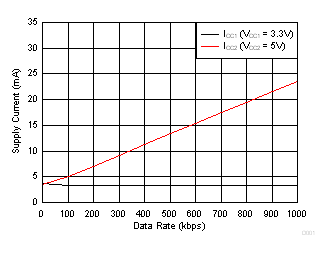TA = 25°C DE = VCC1 RE = GND1
Figure 3. Supply Current Vs Data Rate- No Load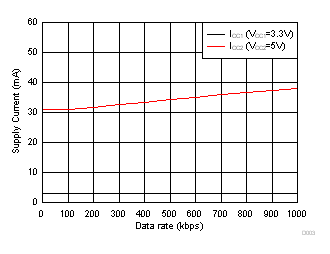TA = 25°C DE = VCC1 RE = GND1 Driver load = 120 ohm || 50 pF Load on R = 15 pF
Figure 5. Supply Current Vs Data Rate - with 120 Ω || 50 pf Load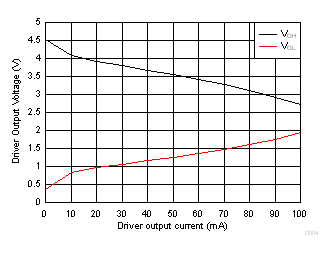DE = VCC1 D = GND1 VCC1 = 3.3 V VCC2 = 5 V TA = 25°C
Figure 7. Driver Output Voltage Vs Driver Output Current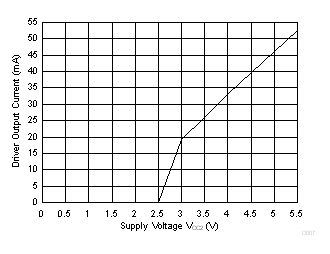TA = 25°C RL = 54 ohm DE = D = VCC1
Figure 9. Driver Output Current Vs Supply Voltage (VCC2)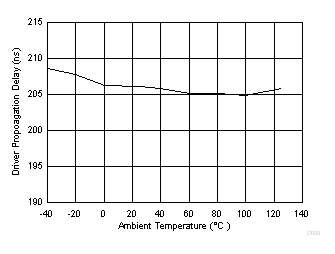VCC1 = 3.3 V VCC2 = 5 V
Figure 11. Driver Propagation Delay vs Temperature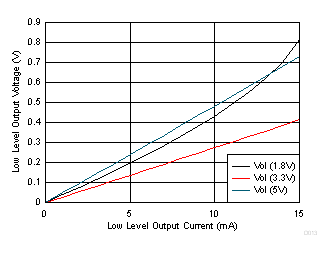TA = 25°C
Figure 13. Receiver Buffer Low Level Output Voltage Vs Low Level Output Current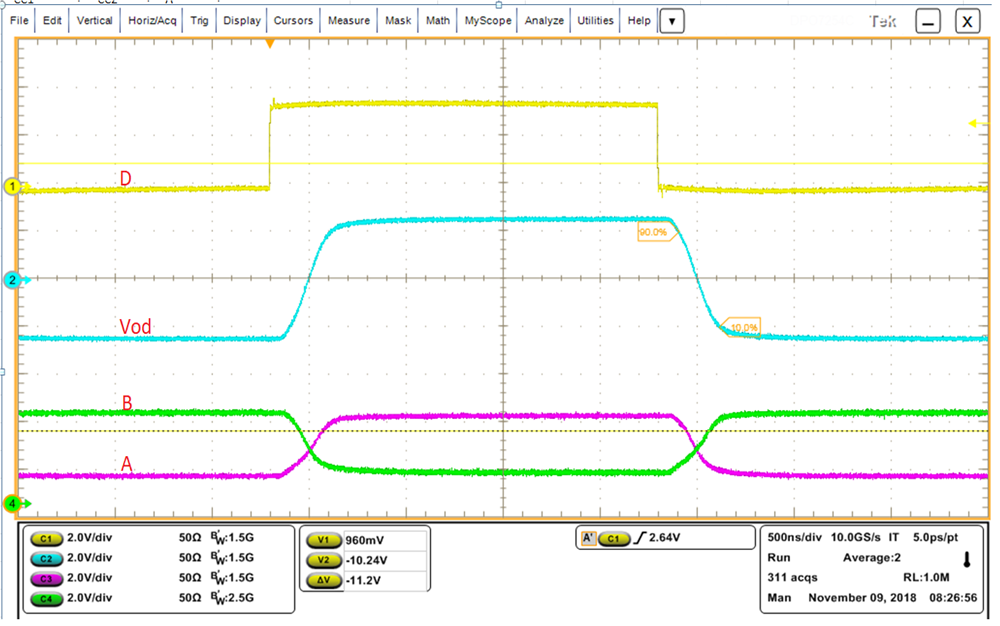VCC1 = 3.3 V VCC2 = 5 V DE = VCC1 TA = 25°C
Figure 15. Driver Propagation delay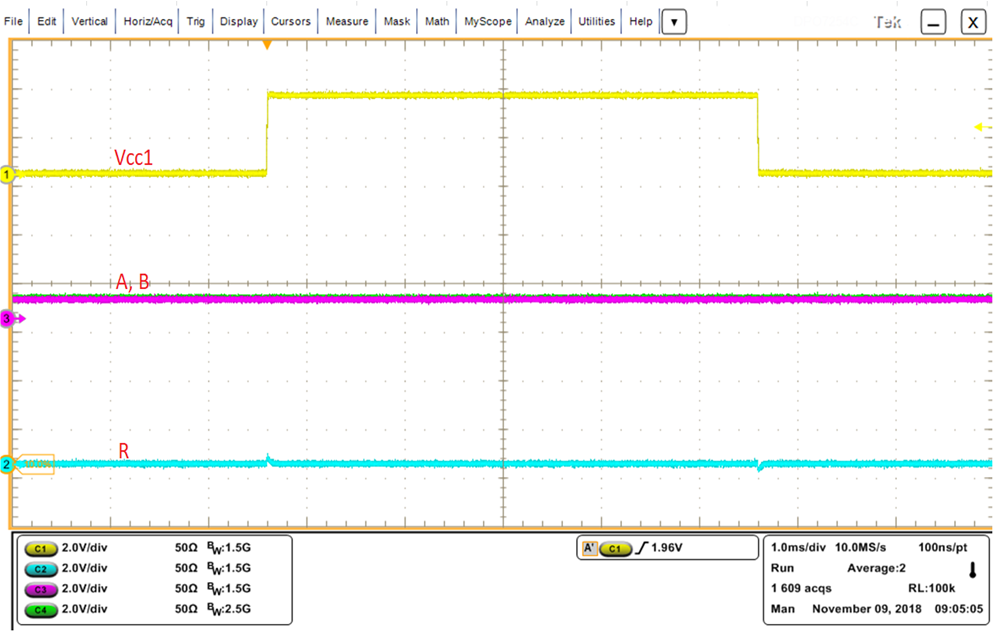Figure 17. VCC1 Power Up / Power down- Glitch Free Behavior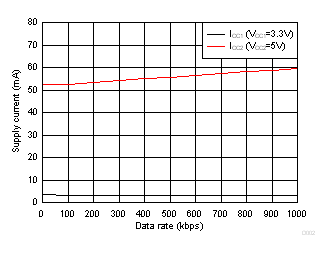TA = 25°C DE = VCC1 RE = GND1 Driver load = 54 ohm || 50 pF Load on R = 15 pF
Figure 4. Supply Current Vs Data Rate- with 54 Ω || 50 pf LoadDE = VCC1 D = GND1 VCC1 = 3.3 V VCC2 = 5 V TA = 25°C
Figure 6. Driver Differential Output Voltage Vs Driver Output Current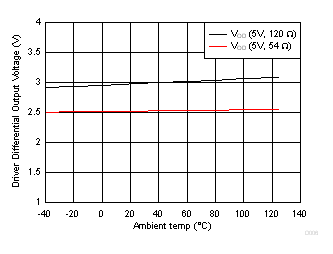Figure 8. Driver Differential Output Voltage Vs Temperature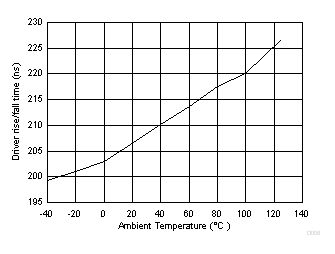VCC1 = 3.3 V VCC2 = 5 V
Figure 10. Driver rise/fall time vs Temperature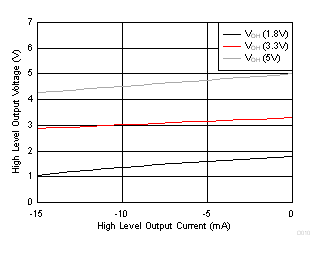TA = 25°C
Figure 12. Receiver Buffer High Level Output Voltage Vs High Level Output Current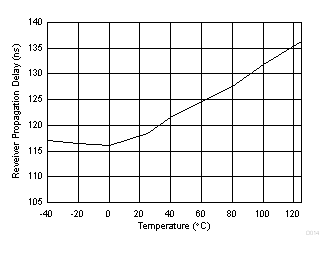VCC1 = 3.3 V VCC2 = 5 V
Figure 14. Receiver Propagation Delay Vs Temperature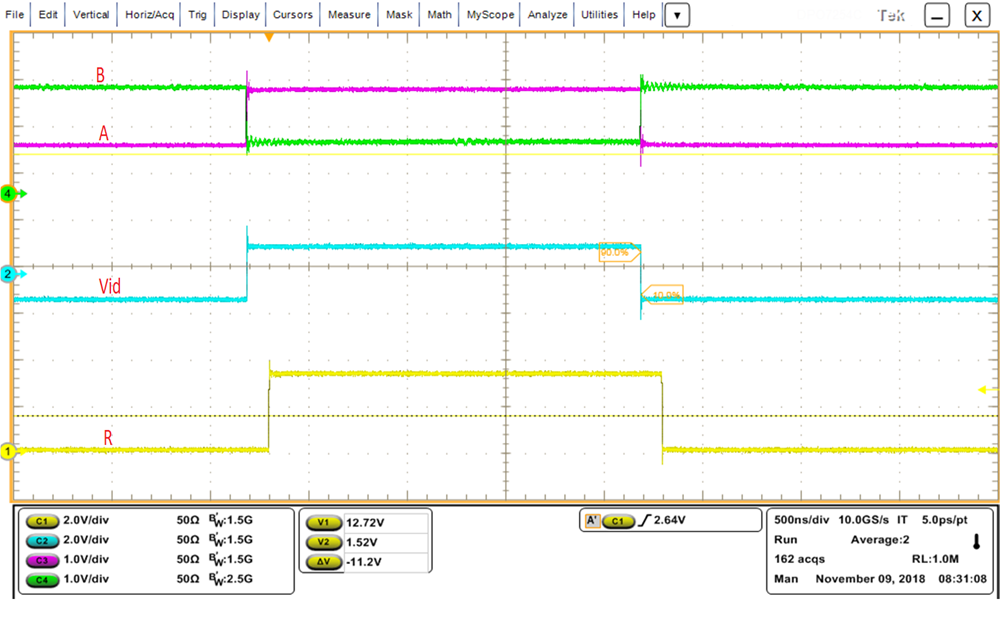VCC1 = 3.3 V VCC2 = 5 V TA = 25°C, DE = GND1 RE = GND1